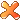## 倒立摆稳定之单节摆

2013年12月14日 发表评论 阅读评论# 力学分析

$$p=(\dfrac{1}{2}d \cos (\theta (t))+x(t),\dfrac{1}{2}d \sin (\theta (t)))$$

## 拉格朗日量 Lagrangian

$$\dfrac{d}{dt}\dfrac{\partial L}{\partial x'(t)}-\dfrac{\partial L}{\partial x(t)}=0$$

$$\left\{\begin{array}{ll}\dfrac{d}{dt}\dfrac{\partial L}{\partial x'(t)}-\dfrac{\partial L}{\partial x(t)}=f(t)\\\dfrac{d}{dt}\dfrac{\partial L}{\partial \theta'(t)}-\dfrac{\partial L}{\partial \theta(t)}=0\end{array}\right.$$

### Mathematica Code### Mathematica Code

SimulatePendulum[2.5 (\[Pi]/2 - \[Theta][t])]

### CDF演示### CDF演示

$$x(t)=0,x'(t)=0,\theta (t)=\dfrac{\pi}{2},\theta ‘(t)=0$$

$$f(t)=k_1x(t)+k_2x'(t)+k_3(\theta (t)-\dfrac{\pi}{2})+k_4\theta ‘(t)$$

$$k_i$$我们这里一般叫做反馈增益。要怎么稳定到平衡点？Mathematica提供了很多控制方程的函数，wolfram里面提到可以用 StateSpaceModel函数来实现非线性的控制方程，它返回的是一个控制模型：### Mathematica Code### Mathematica Code

$$cost function = 1x(t)^2+10x'(t)^2+10\theta (t)^2+100\theta ‘(t)^2+1 f(t)^2$$### CDF演示

gains = LQRegulatorGains[
N[model], {DiagonalMatrix[{1000, 10, 10, 100}], {{1}}}] // First

SimulatePendulum[controlforce + bumps[t]]

# 写完收工

so~断断续续写了一周，总算写的差不多了，这篇就先这样吧，不知道有没有一开始不知道mathematica的同学突然对mathematica感兴趣起来了呢？仔细看下来的话，其实真的可以发现Mathematica在很多方面的运用是超级强大的！！

http://blog.wolfram.com/2011/01/19/stabilized-inverted-pendulum/

【完】

1. 2014年4月9日02:50 | #1

窝只懂物理…那个动能减势能是拉格朗日量的定义，如果我们定义一个函数叫x(t)，还有它的导数x'(t)，那么拉格朗日量就是这两个函数构成，它对时间积分得到作用量，这时候作用量与t无关了因为t被积分掉了，但是作用量可以看做是x这个函数的函数(其实就是泛函)，我们不先验的知道这个x到底是个啥函数，任意的光滑函数都行，但是在众多函数之中只有一个函数复合真正的运动规律，那就是使得作用量变分为0的那个，这是最小作用量原理的准确描述。不过经典力学没有告诉我们为什么要是变分为0的那个才是符合物理的，但是量子力学有答案，因为量子力学是任意一个函数x，都对应有一个一个作用量S，这个S当然不见得是最小的，但是量子力学是把所有的x按照”权重”求和得到粒子传播的概率振幅，这个权重就是exp(iS/hbar)，hbar是约化普朗克常量，于是就会发现，求和之后只有S变分为0(经典路径)的附近的贡献是叠加的，越远离这个经典路径振荡越厉害，求和抵消了，所以最终的效果是只有经典路径附近概率最大。。。好像一下扯太远了

• 2014年4月9日02:51 | #2

S[ x ]。。。怎么变成奇怪的字体了

• 2014年4月9日14:24 | #3

多说留言是这样的，所以我们一般都是s【i】这么敲。。唉。。后面量子力学分析那个第一次听说。。。有点明白，但是又好像不怎么明白。。

2. 2015年1月23日23:32 | #4

1/2mv^2是动能吧

• 2015年1月24日00:58 | #5

嗯，手滑，多谢指正。。其实这种远古博文平时没人看。。

3. 2015年1月23日23:41 | #6

如果要考虑边界条件应该怎么办？因为那个杆一旦倒在车上(认为杆和车完全非弹性碰撞使得相对速度为0)就不会再起来了啊

• 2015年1月24日00:59 | #7

什么意思？杆的极限位置就是水平状态？

• 2015年1月24日22:12 | #8

对，就比如第一次不施加外力的情况，gif中显示杆从水平面下转了回来，但是实际上这是不可能的，应该是杆一接触水平面就立刻静止才对

4. 2020年2月7日16:30 | #9

有一个问题啊，楼主，在计算L的时候，摆杆的势能里是不是少一个g（重力加速度）？